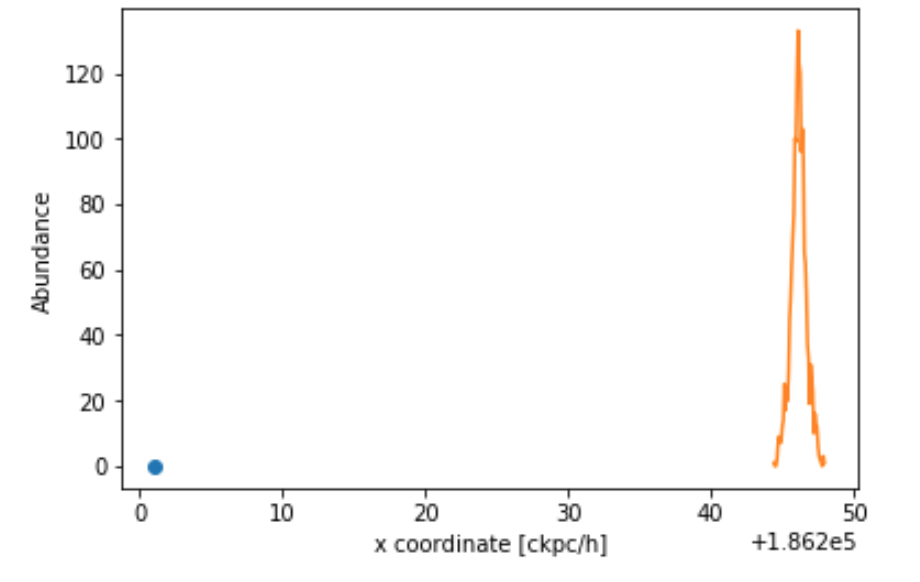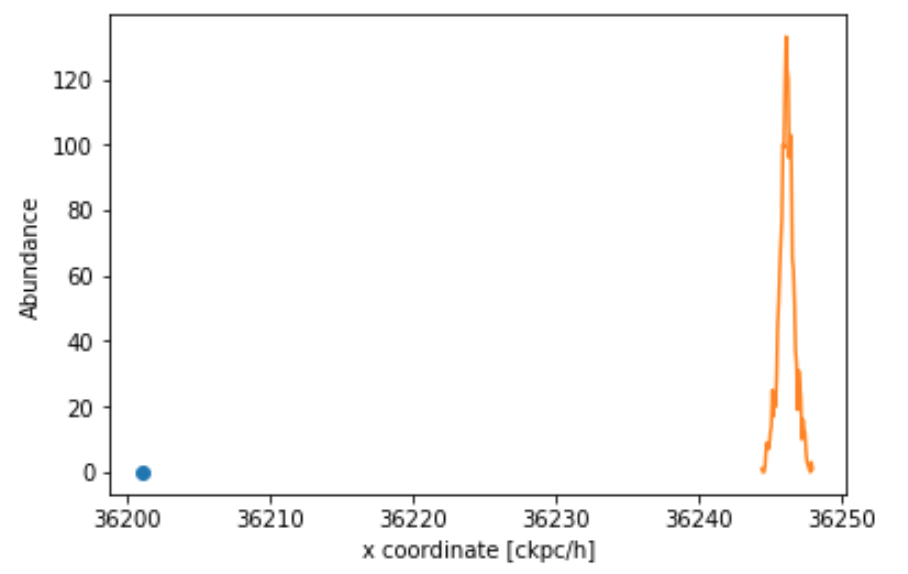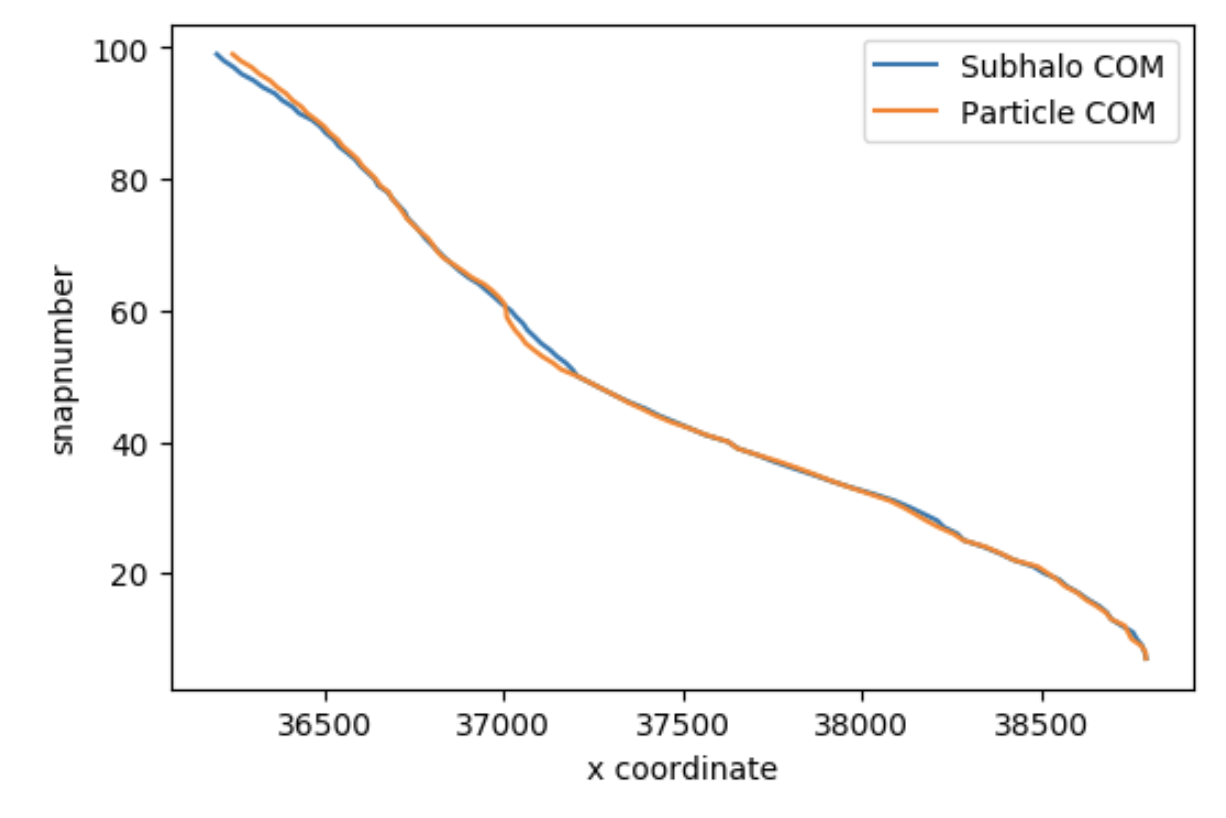### Inconsistencies between particle/halo coordinates?Christopher Marsden
• 12 Mar

Hi. If I compare subhalo particle coordinates with subhalo catalog coordinates e.g.:

`````` subhalo_id = 

for j, snapnum in enumerate(tree['SnapNum']): # Look back through the tree
SubfindID = tree['SubfindID'][j]
particle_data = il.snapshot.loadSubhalo(basePath, snapnum, SubfindID, 'stars', fields=['Coordinates', 'Velocities', 'Masses'])
# Now, compare the particle coordinate data and the catalog_data['SubhaloCM']
``````

Over one axis (in this case x), I get (apologies for the poor quality of the plot):The blue dot represents the x location of the catalog_data subhalo centre of mass. This should surely be centred on the stellar particles, or at least be much close?

Is there some difference in coordinate system that I am unaware of coming into play here?Dylan Nelson
• 13 Mar

This should be due to the periodic boundaries, see e.g. this older thread.Christopher Marsden
• 1
• 13 Mar

This does not explain the issue here. Having corrected for the box size (coordinates modulo boxsize/2), the plot is the same:Are you sure I am comparing the same haloes here? Could this be an instance of the halo at a different time, especially considering it is so close?Dylan Nelson
• 14 Mar

What simulation / basePath?Christopher Marsden
• 14 Mar

basePath = '../sims.TNG/TNG300-1/output/'

It's possible I've made a simple mistake somewhere...Christopher Marsden
• 15 Mar

I believe I've fixed the problem here. I'm not sure what I was doing wrong, but the centre of masses seem consistent now, when plotted against the snapshot number. Full code to produce this follows. Some error checking was required as some snapshots don't seem to return the keys. I hope this can help sombody.``````basePath = '../sims.TNG/TNG300-1/output/'

subhalo_id = 953771

box_size = 75000 # Box size

CMs = tree['SubhaloCM']
snaps = tree['SnapNum']

ParticleCMs = []
invalid_indexes = []

for j, snapnum in enumerate(tqdm(tree['SnapNum'])): # Look back through the tree

SubfindID = tree['SubfindID'][j]
particle_data = il.snapshot.loadSubhalo(basePath, snapnum, SubfindID, 'stars', fields=['Coordinates', 'Velocities', 'Masses'])

if 'Coordinates' not in particle_data:
invalid_indexes.append(j)
continue

test_data = particle_data['Coordinates']
test_weights = particle_data['Masses']/np.sum(particle_data['Masses']) # Normalized

mass_centre = np.average(test_data, axis=0, weights = test_weights)  #catalog_data['SubhaloCM']

ParticleCMs.append(mass_centre)

CMs = np.delete(CMs, invalid_indexes, axis = 0)
snaps = np.delete(snaps, invalid_indexes)

plt.figure(dpi=100)
plt.plot(CMs[:, 0]%box_size, snaps, label='Subhalo COM')
plt.plot(np.array(ParticleCMs)[:, 0]%box_size, snaps, label = 'Particle COM')
plt.xlabel("x coordinate")
plt.ylabel("snapnumber")
plt.legend()
plt.show()
``````Dylan Nelson
• 15 Mar

Thanks for the follow-up, I suspect a (snapshot) mismatch between different arrays. Whenever you're appending things onto a python list, good to be very careful about how things are kept in sync. As you point out, a given merger tree does not need to contain all snapshot number in sequential order (some could be skipped). Also, a given subhalo does not need to have any stars, so you could check the return of `loadSubhalo` as you do or with the `count` attribute.

• Page 1 of 1